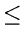Next: 4.9 Programming Assignments Up: 4.8 Problems Previous: 4.8.2 Binary Search Trees

## 4.8.3 Splay Trees

1.
Complete the proof of the following lemmas in the amortized analysis of splay trees:
(a)
If the ith splaying step is a zig-zag step or a zag-zig step at node x, then its amortized complexity ai satisfies ai2(ri(x) - ri - 1(x)).
(b)
If the ith splaying step is a zig step or a zag step at node x, then its amortized complexity ai satisfies ai1 + (ri(x) - ri - 1(x)).
2.
Define a rank function r(x) for the nodes of any binary tree as follows: If x is the root, then r(x) = 0; If x is the left child of y, then r(x) = r(y) - 1; If x is the right child of y, then r(x) = r(y) + 1. Define the credit balance of a tree during a traversal to be the rank of the node being visited. For several binary trees, determine the ranks at each node and prepare a table showing the actual cost, credit balance, and amortized cost (in edges traversed) of each step of an inorder traversal.
3.
Generalize the amortized analysis for incrementing four digit binary integers to n digit binary integers.
4.
A sequence of n operations is performed on a data structure. The ith operation costs i if i is an exact power of 2, and 1 otherwise. Use a suitable credit function and compute the amortized cost per operation.
5.
Keys 1, 2,..., n are inserted in that order into an empty splay tree, using splay insertions. What will be the resulting tree? Also write down the splay tree that results when the key n is deleted, using splay deletion.
6.
Consider a sequence of m accesses (search operations) to an n-node splay tree. Show that the total running time of this sequence of operations is O((m + n)log n + m).Next: 4.9 Programming Assignments Up: 4.8 Problems Previous: 4.8.2 Binary Search Trees
eEL,CSA_Dept,IISc,Bangalore